0
134

Reasoning Tables and Number Series is one of the most important topics in the CMAT, and also it is an important section. One can utilize this article which consists of the most important questions regarding Reasoning Tables and Number Series. Cracku provides you with the Top 20 very Important Reasoning Tables and Number Series Questions for CMAT based on the questions asked in previous exam papers. Click on the link below to download the Reasoning Tables and Number Series Questions for CMAT PDF with detailed answers.

Question 1: Study the given pattern carefully and select the number that can replace the question mark (?) in it.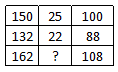a) 29

b) 27

c) 30

d) 24

Solution:

Along the rows, the second number is obtained by dividing the first number by 6 or dividing the third number by 4 as shown below

$\frac{150}{6}=25$   or    $\frac{100}{4}=25$

$\frac{132}{6}=22$   or    $\frac{88}{4}=22$

Similarly, the required number = $\frac{162}{6}=27$

Hence, the correct answer is Option B

Instructions

In the following questions, select the missing number from the given responses.

Question 2: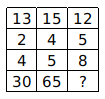a) 64

b) 69

c) 65

d) 68

Solution:

13 $\times$ 2 + 4 = 30

15 $\times$ 4 + 5 = 65

12 $\times$ 5 + 8 = 68

Question 3: Select the missing number from the given responses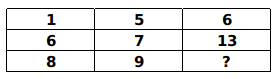a) 17

b) 1

c) 19

d) 7

Solution:

From the table along the rows,

1 + 5 = 6

6 + 7 = 13

Third number is equal to first number and second number along the rows.

Similarly along the third row,

The missing number = 8 + 9 = 17

Hence, the correct answer is Option A

Question 4: Select the missing number from the given responses.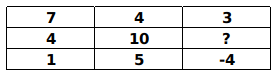a) -6

b) 1

c) -1

d) 14

Solution:

From the table along the rows,

7 – 4 = 3

1 – 5 = -4

Difference between the first number and second number along the rows is equal to the third number.

Let the missing number = a

Similarly along the second row,

4 – 10 = a

$\Rightarrow$  a = -6

$\therefore\$The missing number = -6

Hence, the correct answer is Option A

Question 5: Select the missing number from the given responses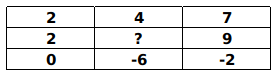a) 2

b) 10

c) 7

d) 11

Solution:

From the table along the columns,

2 – 2 = 0

7 – 9 = -2

Difference of first number and second number is equal to third number along the columns.

Let the missing number = a

Similarly along the second column,

4 – a = -6

$\Rightarrow$  a = 10

$\therefore\$The missing number = 10

Hence, the correct answer is Option B

Question 6: Select the missing number from the given responses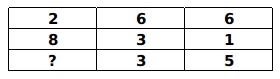a) 10

b) 8

c) -6

d) 6

Solution:

Along the second column and third column,

6 – 3 = 3

6 – 1 = 5

Difference of first two numbers in a column is equal to the third number in the column.

Let the missing number = a

Similarly from the first column,

2 – 8 = a

$\Rightarrow$  a = -6

$\therefore\$The missing number = -6

Hence, the correct answer is Option C

Question 7: Select the missing number from the given responses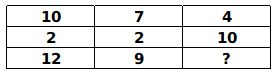a) 21

b) 14

c) 6

d) 3

Solution:

From the table along the columns,

10 + 2 = 12

7 + 2 = 9

Sum of first number and second number is equal to third number along the columns.

Let the missing number = a

Similarly along the third column,

4 + 10 = a

$\Rightarrow$  a = 14

$\therefore\$The missing number = 14

Hence, the correct answer is Option B

Question 8: Select the missing number from the given responses.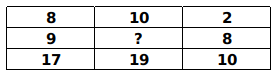a) 1

b) 9

c) -1

d) -9

Solution:

From the table along the columns,

8 + 9 = 17

2 + 8 = 10

Sum of the top two numbers is equal to the bottom number in a column.

Let the required number = a

Similarly, 10 + a = 19

$\Rightarrow$  a = 9

$\therefore\$The required number = 9

Hence, the correct answer is Option B

Question 9: Select the missing number from the given responses: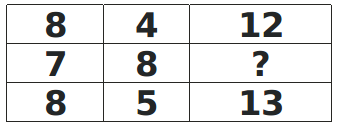a) 1

b) -1

c) 15

d) 4

Solution:

From the table along the columns,

8 + 4 = 12

8 + 5 = 13

Sum of first number and second number is equal to third number along the column.

From the second column, the required number = 7 + 8 = 15

Hence, the correct answer is Option C

Question 10: Select the missing number from the given responses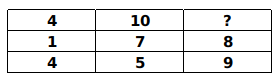a) 6

b) 14

c) 1

d) 17

Solution:

From the table,

1 + 7 = 8

4 + 5 = 9

Along the rows, sum of first number and second number is equal to the third number.

Similarly, the required number = 4 + 10 = 14

Hence, the correct answer is Option B

Question 11: Select the missing number from the given responses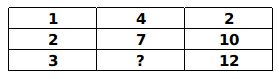a) 3

b) 9

c) -3

d) 11

Solution:

Along the columns,

$1+2=3$

$2+10=12$

Sum of the first and second number is equal to the third number along the columns.

Similarly, the required number $=4+7=11$

Hence, the correct answer is Option D

Question 12: Study the given pattern carefully and select the number that can replace the question mark (?) in it?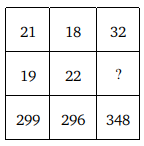a) 14

b) 24

c) 30

d) 28

Solution:

$\frac{299 + 100}{21} = \frac{399}{21}$ = 19
$\frac{296 + 100}{18} = \frac{396}{18}$ = 22
Similarly,
$\frac{348 + 100}{32} = \frac{448}{32}$ = 14
$\therefore$ The correct answer is option A.

Question 13: Study the given pattern carefully and select the number that can replace the question mark (?) in it.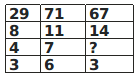a) 4

b) 3

c) 5

d) 6

Solution:

$\frac{29 + 3}{8} = \frac{32}{8} = 4$
$\frac{71 + 6}{11} = \frac{77}{11} = 7$
$\frac{67 + 3}{14} = \frac{70}{14} = 5$
$\therefore$ The correct answer is option C.

Question 14: Study the given pattern carefully and select the number that can replace the question mark (?) in it.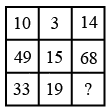a) 27

b) 28

c) 29

d) 25

Solution:

The logic here is

$\left(10-3\right)\times2=14$

$\left(49-15\right)\times2=68$

Along the rows, twice the difference of first number and second number is equal to third number.

Similarly from the third row, the required number = $\left(33-19\right)\times2=28$

Hence, the correct answer is Option B

Question 15: Study the given pattern carefully and select the number that can replace the question mark (?) in it.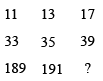a) 217

b) 203

c) 199

d) 195

Solution:

The logic here is

Along first row

11 + 2 = 13

13 + 4 = 17

Along second row

33 + 2 = 35

35 + 4 = 39

Along the rows, second number is the sum of first number and 2, third number is the sum of second number and 4.

Similarly,

Along the third row

189 + 2 = 191

191 + 4 = 195

$\therefore\$The required number = 195

Hence, the correct answer is Option D

Question 16: Study the given pattern carefully and select the number that can replace the question mark (?) in it.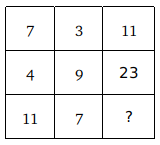a) 71

b) 77

c) 63

d) 59

Solution:

(7 $\times$ 3) – (7 + 3) = 21 – 10 = 11
(4 $\times$ 9) – (4 + 9) = 36 – 13 = 23
Similarly,
(11 $\times$ 7) – (11 + 7) = 77 – 18 = 59
$\therefore$ The correct answer is option D.

Question 17: Study the given pattern carefully and select the number that can replace the question mark (?) in it.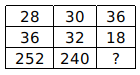a) 268

b) 166

c) 164

d) 162

Solution:

(28$\times 36)\div 4 = 1008/4 = 252$
(30$\times 32)\div 4 = 960/4 = 240$
Similarly,
(36$\times 18)\div 4 = 648/4 = 162$
$\therefore$ The correct answer is option D.

Question 18: Study the given pattern carefully and select the number that can replace the question mark (?) in it.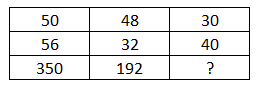a) 150

b) 145

c) 180

d) 140

Solution:

(50 $\times 56) \div 8 = 2800/8 = 350$
(48 $\times 32) \div 8 = 1536/8 = 192$
Similarly,
(30 $\times 40) \div 8 = 1200/8 = 150$
$\therefore$ The correct answer is option A.

Question 19: Study the given pattern carefully and select the number that can replace the question mark(?) in it.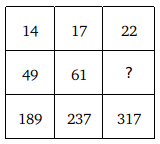a) 83

b) 71

c) 79

d) 81

Solution:

14 $\times$ 4 – 7 = 49, 49 $\times$ 4 = 196 – 7 = 189
17 $\times$ 4 – 7 = 61, 61 $\times$ 4 = 244 – 7 = 237
Similarly,
22 $\times$ 4 – 7 = 81, 81 $\times$ 4 = 324 – 7 = 317
$\therefore$ The correct answer is option D.

Question 20: Study the given pattern carefully and select the number that can replace the question mark (?) in it.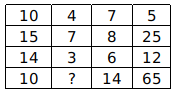a) 14

b) 16

c) 10

d) 18

(15 – 14) $\times 10 = 1 \times 10$ = 10
(8 – 6) $\times 7 = 2 \times 7$ = 14
(25 – 12) $\times 5 = 13 \times 5$ = 65
(7 – 3) $\times 4 = 4 \times 4$ = 16
$\therefore$ The correct answer is option B.